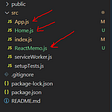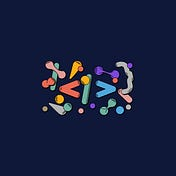# N-Queens

`Input: n = 4Output: [[".Q..","...Q","Q...","..Q."],["..Q.","Q...","...Q",".Q.."]]Explanation: There exist two distinct solutions to the 4-queens puzzle as shown above`
`Input: n = 1Output: [["Q"]]`
• `1 <= n <= 9`
`class Solution(object): def solveNQueens(self, n): “”” :type n: int :rtype: List[List[str]] “”” self.res = []   def dfs(nums, index, ans): if index == len(nums): self.res.append(ans) return   for i in range(len(nums)): nums[index] = i if isValid(nums, index): temp = “.”*len(nums)  dfs(nums, index+1, ans + [temp[:i]+”Q”+temp[i+1:]])  def isValid(nums, index):  for i in range(index): if nums[i] == nums[index] or abs(nums[i] — nums[index]) == index — i: return False return True  dfs([-1]*n, 0, []) return self.res`

--

--

--

## More from Newbie Developer

Love podcasts or audiobooks? Learn on the go with our new app.

## What is React Memo and How to use it## Deep Learning in JavaScript (Part 3)## 5 Benefits of using Next Js along with React JS | Copperchips## Build Your First Discord GIF BOT and Deploy## An Introduction to the Web3 Stack for Beginners## How To Create Custom Event Listeners in JavaScript## Igpay Atinlay, Hoisting, and JavaScript Standards## Around the Metaverse## Draftkings 2020 Review